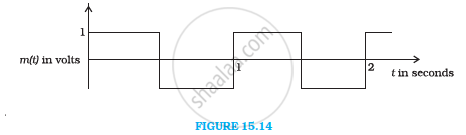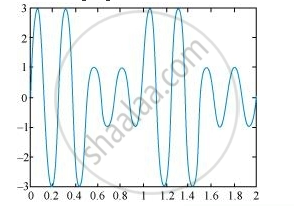Share

# A modulating signal is a square wave. Sketch the amplitude modulated waveform - CBSE (Science) Class 12 - Physics

#### Question

A modulating signal is a square wave, as shownThe carrier wave is given by  c(t) = 2 sin (8pit) "volts"

(i) Sketch the amplitude modulated waveform

(ii) What is the modulation index?

#### Solution

It can be observed from the given modulating signal that the amplitude of the modulating signal, Am = 1 V

It is given that the carrier wave c (t) = 2 sin (8πt)

∴Amplitude of the carrier wave, Ac = 2 V

Time period of the modulating signal Tm = 1 s

The angular frequency of the modulating signal is calculated as:

omega_m = 2pi/T_m

= 2pi "rad" s^(-1)  ... (1)

The angular frequency of the carrier signal is calculated as:

omega_c = 8pi "rad" s^(-1)  ... (ii)

From equations (i) and (ii), we get:

mega_c = 4omega_m

The amplitude modulated waveform of the modulating signal is shown in the following figure.(ii)Modulation index, m = A_m/A_c = 1/2 = 0.5

Is there an error in this question or solution?

#### APPEARS IN

NCERT Solution for Physics Textbook for Class 12 (2018 to Current)
Chapter 15: Communication Systems
Q: 6 | Page no. 530

#### Video TutorialsVIEW ALL 

Solution A modulating signal is a square wave. Sketch the amplitude modulated waveform Concept: Amplitude Modulation.
S